# WBJEE 2015 Physics Paper with Solutions

WBJEE 2015 Physics paper is the best resource to gain insight into the examination. WBJEE 2015 exam is one of the most sought out examinations by the students of West Bengal who are aspiring to get admission in prestigious institutions. The solutions uploaded at BYJU’S will enable the students to divide and plan their resources in an optimal way. Practising these questions will help the students to achieve their goals. Solving these papers will help the candidates in understanding the question paper pattern, improve their time management and also help them identify their strengths and weaknesses.

### WBJEE 2015 - Physics

Question 1: Two particles of mass m1 and m2 approach each other due to their mutual gravitational attraction only. Then

1. a. accelerations of both the particles are equal
2. b. acceleration of the particle of mass m1 is proportional to m1
3. c. acceleration of the particle of mass m1 is proportional to m2
4. d. acceleration of the particle of mass m1 is inversely proportional to m1

Solution:

According to question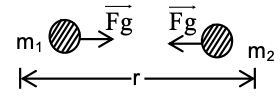Two masses are approaching each other at that time distance between them is r. Now according to Newton’s law of gravitation,

Fg = Gm1m2 / r2 (force of attraction)

(Equal and opposite)

Newton’s 2nd law of motion -: F = ma

a = F/m

Here a and F are vector quantities.

For a of mass m1, a1 = Fg / m1 = [Gm1m2 / r2] / m1 = Gm2 / r2

For a of mass m2, a2 = Fg / m2 = Gm1 / r2

G = 6.67 × 10–11 [N – m2] / [kg2] and r → const. (at any time)

a1 ∝ m2 and a2 ∝ m1

Question 2: Three bodies of the same material and having masses m, m and 3m are at temperatures 40°C, 50°C and 60°C respectively. If the bodies are brought in thermal contact, the final temperature will be

1. a. 45°C
2. b. 54°C
3. c. 52°C
4. d. 48°C

Solution:

According to the law of conservation of energy,

Energy lost = Energy gained

Let the final temperature be T.

Let the specific heat capacity of the material be C.

Then,

mcT1 + mcT2 + 3mcT3 = (mc + mc + 3mc) T

mT1 + mT2 + 3 mT3 = (m + m + 3m) T

mT1 + mT2 + 3mT3 = 5mT

T1 + T2 + 3T3 = 5T

T = [T1 + T2 + 3T3] / 5

= [40 + 50 + 3 * 60] / 5

T = 270 / 5 = 54º C

Question 3: A satellite has kinetic energy K, potential energy V and total energy E. Which of the following statements is true?

1. a. K = –V / 2
2. b. K = V / 2
3. c. E = K / 2
4. d. E = –K / 2

Solution:

The kinetic energy of the satellite is given as:

K = GMm / 2r .............(i)

Similarly, the potential energy is given as;

V = –GMm/r .............(ii)

The total energy of the satellite is given as:

E = K + V

E = [GMm / 2r] + [–GMm/r] --- (iii)

From the equations (i), (ii) and (iii)

K = –V / 2

Question 4: An object is located 4 m from the first of two thin converging lenses of focal lengths 2m and 1m respectively. The lenses are separated by 3 m. The final image formed by the second lens is located from the source at a distance of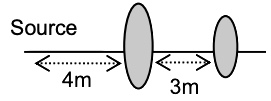1. a. 8.0 m
2. b. 7.5 m
3. c. 6.0 m
4. d. 6.5 m

Solution:

The image obtained by the first lens will act as an object for the second lens.

Here, for the first lens.

u = – 4 m

f1 = 2 m

According to lens formula

(1 / v) - (1 / u) = 1 / f

(1 / v1) - (1 / u1) = 1 / f1

(1 / v1) = (1 / 2) - (1 / 4) = 1 / 4

(1 / v1) = 1 / 4

v1 = 4m

For the second lens,

u2 = 1 m

And f2 = 1m

Again applying the lens formula,

(1 / v2) - (1 / u2) = 1 / f2

(1 / v2) - (1 / 1) = 1 / 1

(1 / v2) = 2

v2 = 1 / 2 = 0.5

Therefore, distance from the object = 4 + 3 + 0.5 = 7.5 m

Question 5: A simple pendulum of length L swings in a vertical plane. The tension of the string when it makes an angle θ with the vertical and the bob of mass m moves with a speed v is (g is the gravitational acceleration

1. a. mv2 / L
2. b. mg cos θ + mv2 / L
3. c. mg cos θ – mv2 / L
4. d. mg cos θ

Solution: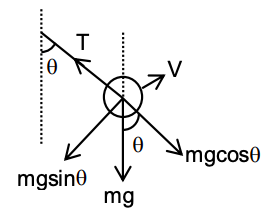From the diagram, we can see that

mg cos θ is the component of mg.

Also, a centripetal force act on the pendulum which is mv2 / L

So, the tension in the string is mg cos θ + mv2 / L.

Question 6: The length of a metal wire is L1 when the tension is T1 and L2 when the tension is T2. Find the unstretched length of the wire.

1. a. [L2 + L2] / 2
2. b. √L1L2
3. c. [T2L1 - T1L2] / [T2 - T1]
4. d. [T2L1 + T1L2] / [T2 + T1]

Solution:

Let the initial length of the metal wire is L

The strain at tension T1 is ΔL1 = L1 – L

The strain at tension T2 is ΔL2 = L2 – L

Suppose, the young’s modulus of the wire is Y

(T1 / A) / (ΔL1 / L) = (T2 / A) / (ΔL2 / L)

Young’s modulus (Y) = stress (σ) / strain (𝜺) = (F / A) / (ΔL / L)

Where A is the area of cross-section of the wire assumes to be same at all the situations.

(T1 / A) (L / ΔL1) = (T2 / A) (L / ΔL2)

T1 / [L1 - L] = T2 / [L2 - L]

T1 (L2 – L) = T2 (L1 – L)

L = [T2L1 - T1L2] / [T2 - T1]

Question 7: The line AA’ is on a charged infinite conducting plane which is perpendicular to the plane of the paper. The plane has a surface density of charge σ and B is a ball of mass m with a like charge of magnitude q. B is connected by a string from a point on the line AA’. The tangent of the angle (θ) formed between the line AA’ and the string is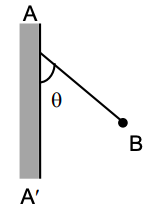1. a. qσ / 2𝝐0mg
2. b. qσ / 4𝛑𝝐0mg
3. c. qσ / 3𝛑𝝐0mg
4. d. qσ / 𝝐0mg

Solution:

The diagram is as follows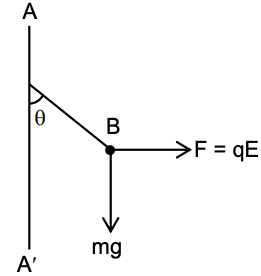The electric field due to the charged infinite conducting sheet is E = σ / 𝝐0.

Now, force (electric force) on the charged ball is F = qE = qσ / 𝝐0

The resultant of electric force and mg balance the tension produced in the string. So, tan θ = Fe / mg = [qσ / 𝝐0] / mg = qσ / 𝝐0mg

Question 8: The current I in the circuit shown is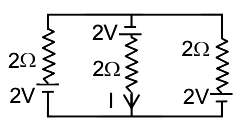1. a. 1.33A
2. b. zero
3. c. 2.00 A
4. d. 1.00 A

Solution:

We first try to find the equivalent emf and internal resistance of the two side branches.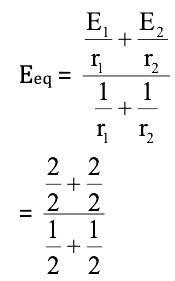= 2V

req = [r1r2] / [r1 + r2] = 1Ω

This equivalent cell is in series with the central branch.

Hence current = [Eeq + E3] / [req + r3]

= [2V + 2V] / [1Ω + 2Ω] = (4 / 3)A = 1.33 A

Question 9: A hollow sphere of external radius R and thickness t (<< R) is made of a metal of density 𝞀, the sphere will float in water if

1. a. t ≤ R / 𝞀
2. b. t ≤ R / 3𝞀
3. c. t ≤ R / 2𝞀
4. d. t ≥ R / 2𝞀

Solution:

The density of the material is 𝞀.

The hollow sphere will float if its weight is less than the weight of the water displaced by the volume of the sphere. This implies the mass of the sphere is less than that for the same volume of water. Now, the mass of spherical shell,

m1 = 4𝛑R2 × t × 𝞀

While the mass of water having the same volume,

m2 = (4 / 3) 𝛑R3 × t × 𝞀g = (4 / 3) 𝛑R3

Where, 𝞀g = density of water = 1 gm/cm3

For the floatation of the sphere,

m1 ≤ m2

4𝛑R2 × t × 𝞀 ≤ (4 / 3) 𝛑R3

t . 𝞀 ≤ R / 3

t ≤ R / 3𝞀

Question 10: A metal wire of circular cross-section has a resistance R1. The wire is now stretched without breaking so that its length is doubled and the density is assumed to remain the same. If the resistance of the wire now becomes R2 then R2 : R1 is

1. a. 1 : 1
2. b. 1 : 2
3. c. 4 : 1
4. d. 1 : 4

Solution:

The wire is stretched to double its length.

l’ = 2 l

The volume in the process remains the same. (Since mass is unchanged, and density remains same)

l’A’ = lA

A’ = lA / l’ = Al / 2l

A’ = A / 2

If the initial resistance was, R = 𝞀l / A

Hence, R’ = 𝞀l’ / A’

R’ = [𝞀 * 2l] / (A / 2) = [4𝞀l / A]

R’ = 4R

R’ / R = 4 / 1 or 4:1

Question 11: Assume that each diode shown in the figure has a forward bias resistance of 50Ω and an infinite reverse bias resistance. The current through the resistance 150Ω is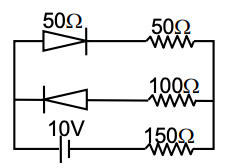1. a. 0.66A
2. b. 0.05A
3. c. zero
4. d. 0.04A

Solution:

Since the positive terminal of the battery is attached to the positive terminal of the upper diode, it is forward bias, and hence conducts current offering 50Ω resistance. Since the positive terminal of the battery is attached to the negative of the lower diode, it is reverse bias, and hence does not conduct current at all. Hence that branch can be ignored in the circuit.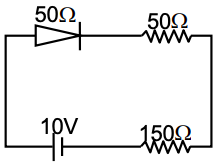Thus, the current flowing in rest of the

Circuit = [10v] / [150Ω + 50Ω + 50Ω] = 0.04 A

Question 12: The r.m.s speed of oxygen is v at a particular temperature. If the temperature is doubled and oxygen molecules dissociate into oxygen atoms, the r.m.s speed becomes

1. a. V
2. b. √2v
3. c. 2v
4. d. 4v

Solution:

The root mean square speed of a gas is given by √(3RT/M)

Where T is the temperature of the gas and M is the molecular weight of the gas.

When oxygen molecules dissociate into oxygen atoms, the molecular weight of gas halves.

Hence,

T’ = 2T

M’ = M / 2

V’/V = √([T’/M’]/[T/M]) = √((2T/M/2)/(T/M))

V’ / V = √4 / 1 = 2

V’ = 2V

Question 13: Two particles, A and B, having equal charges, after being accelerated through the same potential difference enter a region of the uniform magnetic field and the particles describe circular paths of radii R1 and R2 respectively. The ratio of the masses of A and B is

1. a. √R1 / R2
2. b. R1 / R2
3. c. (R1 / R2)2
4. d. (R2 / R1)2

Solution:

Since the charge on both particles is same and they are accelerated through the same voltage, work done by electric field will be the same for both the charges so the increase in kinetic energy will be the same. Assuming that charges started from rest, we have:

(1 / 2)m1v12 = qv = (1 / 2)m2v22

v12 / v22 = m2 / m1

Where V is the potential difference through which the charges are accelerated. The magnetic force only provides the necessary centripetal force for the circular motion :

m1v12 / R1 = Bqv1

m2v22 / R2 = Bqv2

R1 / R2 = m1v1 / m2v2 = √m1 / √m2

m1 / m2 = R12 / R22

Question 14: A large number of particles are placed around the origin, each at a distance R from the origin. The distance of the centre of mass of the system from the origin is

1. a. = R
2. b. ≤ R
3. c. > R
4. d. ≥ R

Solution:

As a large number of particles are situated at a distance R from the origin. If particles are uniformly distributed and make a circular boundary around the origin, then the centre of mass will be at the origin.

While, if the particles are not uniformly distributed then the centre of mass will lie between particle and origin. This implies that the distance between the centre of mass and origin is always less than or equal to R. So, Answer will be ≤ R.

Question 15: A straight conductor 0.1m long moves in a uniform magnetic field 0.1 T. The velocity of the conductor is 15 m/s and is directed perpendicular to the field. The e.m.f. induced between the two ends of the conductor is

1. a. 0.10 V
2. b. 0.15V
3. c. 1.50 V
4. d. 15.00V

Solution:

The EMF induced across a conductor of length l, moving perpendicular to a magnetic field B with velocity V is equal to BVl

EMF induced in given problem = BVl

= 0.1 T × 15 m/s × 0.1 m

= 0.15 V

Question 16: A ray of light is incident at an angle i on a glass slab of refractive index μ. The angle between reflected and refracted light is 90 degree. Then the relationship between i and μ is

1. a. i = tan-1 (1 / μ)
2. b. tan i = μ
3. c. sin i = μ
4. d. cos i = μ

Solution:

Let the angle of reflection and refraction be r1, r2 respectively.

Since the angle between reflected and refracted ray is 90°,

r1 + r2 = 90o

From the law of reflection, r1 = i

From Snell’s law,

sin i = μ sinr2

= μ sin (90° – r1)

sin i = μ cosr1 = μ cos i

tan i = μ

Question 17: Two particles A and B are moving as shown in the figure. Their total angular momentum about the point O is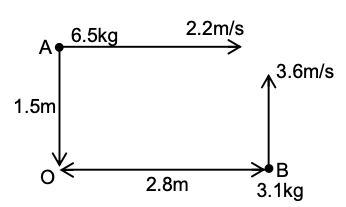1. a. 9.8 kg m2/s
2. b. Zero
3. c. 52.7 kg m2/s
4. d. 37.9 kg m2/s

Solution:

Total angular momentum about 0 is given as,

L = L1 + L2

= m1v1r1 + m2v2r2

= – 6.5 × 2.2 × 1.5 + 3.1 × 3.6 × 2.8

(Considering anticlockwise direction as negative angular momentum)

= – 21.45 + 31 .24 L

= 9.8 kg [m2 / sec]

Question 18: A 20 cm long capillary tube is dipped vertically in the water and the liquid rises up to 10 cm. If the entire system is kept in a freely falling platform, the length of the water column in the tube will be

1. a. 5 cm
2. b. 10 cm
3. c. 15 cm
4. d. 20cm

Solution:

The height raised by the liquid in a capillary tube,

H = 2l cos θ / 𝛒gh

Where H is a rise in the capillary tube.

As in freely falling platform, a body experience weightlessness.

geff = 0

So, the liquid will rise up to a length of the capillary. i.e. height raised by the liquid will be 20 cm.

Question 19: A train is moving with a uniform speed of 33 m/s and an observer is approaching the train with the same speed. If the train blows a whistle of frequency 1000 Hz and the velocity of sound is 333 m/s then the apparent frequency of the sound that the observer hears is

1. a. 1220 Hz
2. b. 1099 Hz
3. c. 1110 Hz
4. d. 1200 Hz

Solution:

Doppler-shifted frequency of sound radiation from a source is given as

f = f0 {[c + v0] / [c - vs]}

c → velocity of sound

v0 → velocity of the observer

vs → velocity of the source

Following the sign convention of the velocity of observer and source

f = 1000 {[333 + 33] / [333 - 33]} Hz

f = 1220 Hz

Question 20: A photon of wavelength 300 nm interacts with a stationary hydrogen atom in the ground state. During the interaction, the whole energy of the photon is transferred to the electron of the atom. State which possibility is correct? (Consider, Planck’s constant = 4 × 10–15 eVs, velocity of light = 3 × 108 m/s, ionization energy of hydrogen = 13.6eV)

1. a. Electron will be knocked out of the atom
2. b. Electron will go to any excited state of the atom
3. c. Electron will go only to the first excited state of the atom
4. d. Electron will keep orbiting in the ground state of an atom

Solution:

The energy of the photon, Eph = hv

Eph = hc / λ [since c = vλ]

Now put the given values,

Eph = [4 * 10-15 * 3 * 108] / [300 * 10-9]

Eph = 4ev

The ionisation energy of hydrogen = 13.6 eV

EIonisation = 13.6 eV

The ionization energy is 13.6 eV which is greater than the energy of photon 13.6 eV > 4 eV, Hence no excitation takes place and electron will keep orbiting in the ground state of the atom.

Question 21: Particle A moves along X-axis with a uniform velocity of magnitude 10 m/s. Particle B moves with uniform velocity 20 m/s along a direction making an angle of 60 with the positive direction of X-axis as shown in the figure. The relative velocity of B with respect to that of A is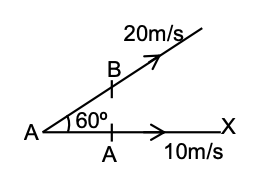1. a. 10 m/s along X-axis
2. b. 10 √3 m/s along Y-axis (perpendicular to X-axis)
3. c. 10 √5 along the bisection of the velocities of A and B
4. d. 30 m/s along negative X-axis

Solution:

Resolving the velocity in horizontal and vertical components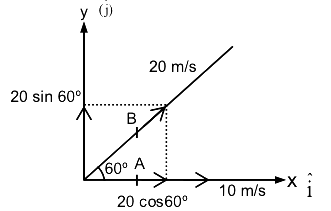The relative velocity of B with respect to that of A is given as VBA,

VBA = VB - VA

= (20 cos 60° i + 20 sin 60° j) – 10 i

= 20 × (1 / 2) i + 20 * (√3 / 2)j – 10i

= 10i + 10 √3 j - 10i

vBA= 10 √3 j

Question 22: When light is refracted from a surface, which of its following physical parameters does not change?

1. a. velocity
2. b. amplitude
3. c. frequency
4. d. wavelength

Solution:

Frequency of the light depends upon the source so it doesn’t change in case of reflection or refraction or polarization.

Question 23: A solid maintained at t1° C is kept in an evacuated chamber at temperature t2°C (t2 >> t1). The rate of heat absorbed by the body is proportional to

1. a. t24 - t14
2. b. (t24 + 273) - (t14 + 273)
3. c. t2 – t1
4. d. t22 - t12

Solution:

1. Answer: (G) Bonus

According to Stefan-Boltzman law

μ = σAT4

Where σ → Stefan – Boltzman constant

tsolid < tsurrounding (given)

Now, the temperature of solid increase and, solid and surrounding will be at equilibrium. So, the rate of emission in case of solid will be proportional at,

Rsolid ∝ T14 [R→ rate of emission]

T1 = (t1 + 273)4

Rsolid ∝ (t1 + 273)4

Rsurrounding & T

Rsurrounding ∝ T24

T2 = (t2 + 273)4

Rsurrounding ∝ (t2 + 273)4

Net Rate ∝ T24 – T14

Net Rate ∝ (t2 + 273)4 – (t1 + 273)4

So, the correct option is this.

Question 24: Block B lying on a table weighs W. The coefficient of static friction between the block and the table is μ. Assume that the cord between B and the knot is horizontal. The maximum weight of the block A for which the system will be stationary is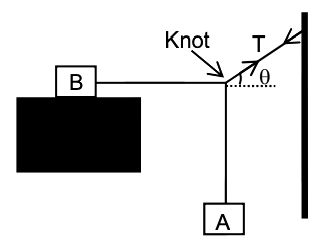1. a. [w tan θ] / μ
2. b. μw tanθ
3. c. μw √1 + tan2 θ
4. d. μw sinθ

Solution: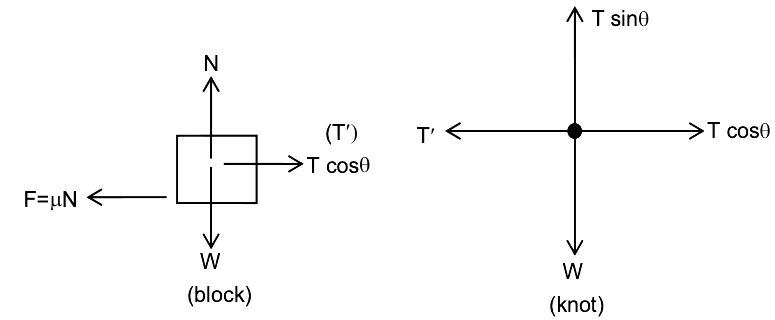The block B is under equilibrium by the action of tension force on it leftwards (say T’), and force of static friction on it leftwards (f').

Hence, f = μN = μW = T’

Consider the force acting on the knot

Balancing the forces on knot horizontally,

T' = T cos θ

Also balancing the forces on knot vertically,

T sin θ = w

w = [T ' / cos θ] . sinθ

w = T’ tan θ

w = μw tan θ

Question 25: The inputs to the digital circuit are shown below. The output Y is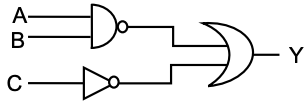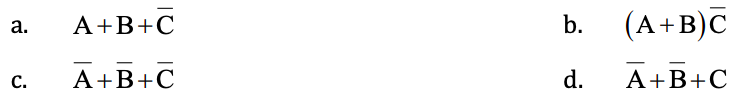Solution:

Here, A, B and C are inputs and Y is output

A and B are inputs to the NAND gate. The output of that gate is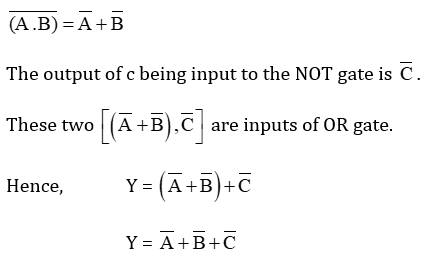Question 26: Two particles A and B having different masses are projected from a tower with the same speed. A is projected vertically upward and B vertically downward. On reaching the ground

1. a. velocity of A is greater than that of B
2. b. velocity of B is greater than that of A
3. c. both A and B attain the same velocity
4. d. the particle with the larger mass attains the higher velocity

Solution: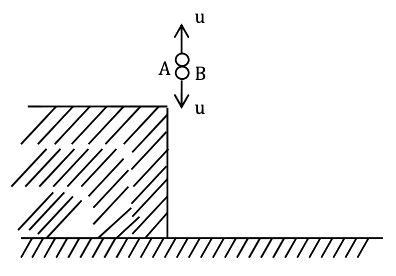Let vA and vB be the final velocities of A and B respectively since the initial velocity of both particles is the same.

So, using the third equation of motion i.e,

v2 = u2 + 2as

vA2 = u2 - 2g (-h) = u2 + 2gh ……….(1)

and vB2 = u2 + 2gh ………(2)

from (1) and (ii)

vA = vB

Question 27: The work function of metals is in the range of 2 eV to 5eV. Find which of the following wavelength of light cannot be used for the photoelectric effect. (Consider, Planck constant = 4 × 10–15 eVs, velocity of light = 3 × 108 m/s)

1. a. 510 nm
2. b. 650 nm
3. c. 400 nm
4. d. 570nm

Solution:

The work function of the metal is given as:

E = hc / λ

So, λ = hc / E

Now,

λmax = hc / Emin

And λmin = hc / Emax

λmin = [4 * 10-15 * 3 * 108] /  = [12 * 10-7] / 5 = 2.4 * 10-7 m

λmin = 240 nm

λmax = [4 * 10-15 * 3 * 108] / 2 = [12 * 10-7] / 2 = 6 * 10-7 m

λmax = 600 nm

So, the wavelength of light that cannot be used for photo electric effect is 650 nm.

Question 28: A thin plastic sheet of refractive index 1.6 is used to cover one of the slits of a double-slit arrangement. The central point on the screen is now occupied by what would have been the 7th bright fringe before the plastic was used. If the wavelength of light is 600 nm, what is the thickness (in μm) of the plastic?

1. a. 7
2. b. 4
3. c. 8
4. d. 6

Solution:

If the central fringe shifts to ‘m’th bright fringe,

(μ – 1) t = mλ

μ = 1.6 and m = 7

λ = 600 nm

t = thickness

(1.6 – 1) t = 7 × 600

t = [7 × 600] / [0.6]

t = 7000 nm

t = 7 μm

Question 29: The length of an open organ pipe is twice the length of another closed organ pipe. The fundamental frequency of the open pipe is 100 Hz. The frequency of the third harmonic of the closed pipe is

1. a. 100 Hz
2. b. 200 Hz
3. c. 300 Hz
4. d. 150 Hz

Solution:

The fundamental frequency of the organ open pipe is given as:-

f0 = v / 2l0 = 100Hz

Similarly, the third harmonic of the closed pipe is given as:

fc = (3 / 4) (v / lc)

It is given that, l0 = 2lc

fc = (3 / 4) (v / [l0 / 2])

= (3 / 2) (v / l0)

f0 = 3 [v / 2l0]

= 3 × 100

f0 = 300 Hz

Question 30: A 5 μF capacitor is connected in series with a 10 μF capacitor. When a 300 Volt potential difference is applied across this combination, the energy stored in the capacitors is

1. a. 15 J
2. b. 1.5 J
3. c. 0.15 J
4. d. 0.10 J

Solution:

Let us assume that,

C1 = 5 μF

C2 = 10 μF

Equivalent capacitances are given as:

Ceq = C1C2 / [C1 + C2]

Ceq = [5 * 10] / [5 + 10] = 50 / 15 = (10 / 3) μF

Hence,

Energy stored in the capacitor is given as:

U = (1 / 2) Ceq V2

= (1 / 2) ([10 / 3] * 10-6) (300)2

U = 0.15 J

Question 31: A cylinder of height h is filled with water and is kept on a block of height h/2. The level of water in the cylinder is kept constant. Four holes numbered 1, 2, 3 and 4 are at the side of the cylinder and at heights 0, h/4 and 3h/4 respectively. When all four holes are opened together, the hole from which water will reach the farthest distance on the plane PQ is the hole no.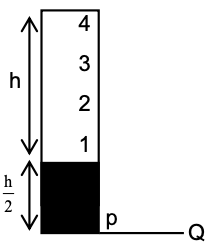1. a. 1
2. b. 2
3. c. 3
4. d. 4

Solution:

We know from Torricelli’s theorem, that the range of the liquid falling from a certain height is given by,

R = 2 * √h (H – h)

Where H is the total height of the container and h is the height where the hole is, For R = Rmax;

dR / dh = 0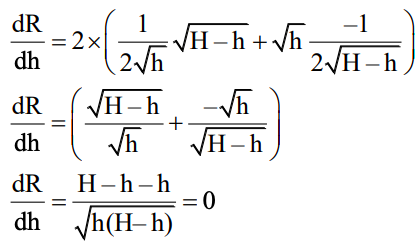H – h – h = 0

H = 2h

For R = Rmax

h = H / 2

Taking PQ as the reference,

H = h + (h / 2) = 3h / 2

So hole must be at height,

(H / 2) = [3h / 2] / 2 = 3h / 4

For hole 1,

h1 = (h / 2) + 0 = h / 2

For hole 2,

h2 = (h / 2) + (h / 4) = 3h / 4

For hole 3,

h3 = (h / 2) + (h / 2) = h

For hole 4,

h4 = (h / 2) + (3h / 4) = 5h / 4

Since, hole 2 is at the H / 2 height required for longest range.

Hole 2 is from which water will reach the farthest distance on the plane PQ.

Question 32: The pressure p, volume v and temperature T for a certain gas are related by p = [AT - BT2] / v, where A and B are constants. The work is done by the gas when the temperature changes from T1 to T2 while the pressure remains constant, is given by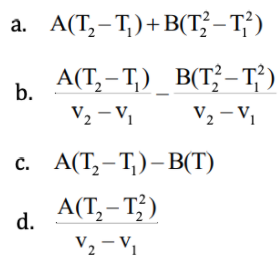Solution:

We have,

P = [AT - BT2] / v

V = [AT - BT2]/p

Work done is given as:-

W = pΔV = p [V2 - V1]

Now,

V2 = [AT2 - BT22]/p

V1 = [AT1 - BT12]/p

W = p[{[AT2 - BT22]/p} - {[AT1 - BT12]/p}]

= p{[AT2 - BT22 - AT1 + BT12]/p}

W = A (T2 - T1) - B(T22 - T12)

Question 33: In the circuit shown below, the switch is kept in position ‘a’ for a long time and is then thrown to position ‘b’. The amplitude of the resulting oscillating current is given by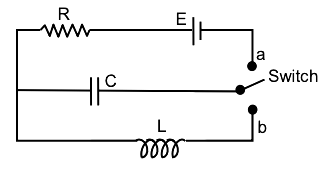1. a. F √L / C
2. b. F / R
3. c. Infinity
4. d. E √C / L

Solution:

The charge through the capacitor is given as :

q0 = CE

Where C is capacitance and E is the potential difference.

Now by conservation of energy,

Energy stored by capacitor = Energy stored by inductor

q02/2c = (1/2) LI02

C2 E2/2C = (1 / 2) LI02

I0 = E √(C/L)

Question 34: A charge q is placed at one corner of a cube. The electric flux through any of the three faces adjacent to the charge is zero. The flux through any one of the other three faces is

1. a. [q / 3𝝐0]
2. b. [q / 6𝝐0]
3. c. [q / 12𝝐0]
4. d. [q / 24𝝐0]

Solution:

Consider a cube as this charge is at the centre so, this cube for which the charge is at the corner becomes 1 / 8. As we know from Gauss Law, the total flux produced by a charge is,

Φ0 = Qencl / 𝝐0

In this amount, 1 / 8th part goes from the cube we are considering. So, flux from the given cube is

Φ0 = Qenclosed / 8𝝐0 = q / 8𝝐0

Therefore, flux passing from the three surfaces in contact with the charges is zero and the flux passes only from the remaining faces. Because of the symmetry, the flux from the remaining three faces will be equal. Therefore, flux passing from each of these faces is;

Φ = [q / 24𝝐0]

Question 35: Two cells A and B of e.m.f 2V and 1.5V respectively, are connected as shown in a figure through an external resistance 10Ω. The internal resistance of each cell is 5Ω. The potential difference EA and EB across the terminals of the cells A and B respectively are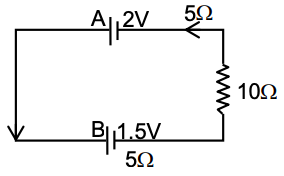1. a. EA = 2.0V, EB = 1.5V
2. b. EA = 2.12V, EB = 1.375V
3. c. EA = 1.875V, EB = 1.625V
4. d. EA = 1.875V, EB = 1.375V

Solution:

Let i be the current flowing through the circuit on applying KVL,

i = [2 –1.5] / 20 = (1 / 40)A

The potential difference through battery A is given as,

EA = 2 – ir = 2 – (1 / 40) * 5 = 1.875 V

Similarly, potential difference through B is given as,

EB = 1.5 + ir = 1.625 v

Question 36: Two charges +q and –q are placed at a distance ‘a’ in a uniform electric field. The dipole moment of the combination is cos θi + sin θj, where θ is the angle between the direction of the field and the line joining the two charges. Which of the following statement(s) is/are correct?

1. a. The torque exerted by the field on the dipole vanishes
2. b. The net force on the dipole vanishes
3. c. The torque is independent of the choice of coordinates
4. d. The net force is independent of ‘d’.

Solution:

1. Answer: (b, c, d)

The net force on a dipole in a uniform electric field is zero because opposite and equal forces on both charges of dipole cancel each other but the torque is given as T = PE sin θ

Where θ is the angle between the electric field and the dipole.

Now, torque is only zero when the dipole is kept along the electric field.

Question 37: Find the right condition(s) for Fraunhofer diffraction due to a single slit.

1. a. Source is at infinite distance and the incident beam has converged at the slit.
2. b. Source is near to the slit and the incident beam is parallel.
3. c. Source is at infinity and the incident beam is parallel.
4. d. Source is near to the slit and the incident beam has converged at the slit.

Solution:

1. Answer: (b, c)

In Fraunhofer diffraction, the source has to be kept at an infinite distance effectively from the slit because then only the incident rays are parallel. This can be achieved if

(i) Point source is kept at the focus of a converging lens

(ii) Point source is at infinite distance

Question 38: A conducting loop in the form of a circle is placed in a uniform magnetic field with its plane perpendicular to the direction of the field. An e.m.f. will be induced in the loop if

1. a. It is translated parallel to itself.
2. b. It is rotated about one of its diameters.
3. c. It is rotated about its own axis which is parallel to the field.
4. d. The loop is deformed from the original shape.

Solution:

1. Answer: (b, d)

Emf will be induced in the loop only when flux changes. Now, if the loop is translated parallel to itself then there is no flux change.

Hence option A is not correct.

If the loop is rotated about one of its diameters then the flux passing through loop changes so, B is the correct option.

If the axis is parallel to the field then again there will be no change in flux and hence no emf will be induced in the loop.

If the loop is deformed then obviously its area will change and hence the flux flowing through it will also change.

So, the correct option is (B) and (D)

Question 39: A circular disc rolls on a horizontal floor without slipping and the centre of the disc moves with a uniform velocity v. Which of the following values the velocity at a point on the rim of the disc can have?

1. a. v
2. b. –v
3. c. 2 v
4. d. zero

Solution:

1. Answer: (c, d)

At each point on the rim, the velocity is tangent due to circular motion and horizontal to the ground due to linear motion of the centre of mass of the disc. So, the resultant velocity will never be v or –v.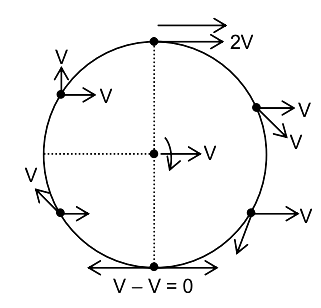It is clear from the figure that at a topmost position on the rim, the velocity is 2v and at the lowermost position, the velocity is 0.

So, the correct options are C and D.

Question 40: Consider two particles of different masses. In which of the following situations the heavier of the two particles will have smaller de Broglie wavelength?

1. a. Both have a free fall through the same height
2. b. Both move with the same kinetic energy.
3. c. Both move with the same linear momentum
4. d. Both move with the same speed

Solution:

1. Answer: (a, b, d)

De Broglie wavelength (λ), it can be given as;

λ = h/p = h/mv = h/√(2mE)

If both particles fall freely from the same height, then

v = √(2gh)

So, De Broglie wavelength

λ = h/m√(2gh)

So, λ ∝ (1/m) for the same v

From the formula of De Broglie wavelength, it can be seen that option B and option D are correct.

### WBJEE 2015 Physics Question Paper with Solutions